# Cos 423 Problem Set No. 5-revised Due Wed. April 23, 2003 Spring 2003

 COS 423 Problem Set No. 5-revised Due Wed. April 23, 2003 Spring 2003 Collaboration Allowed Let G be a connected, undirected graph with real-valued edge weights, let r be a distinguished vertex of G, and let k be an integer. A k-tree of G is a spanning tree of G in which vertex r has degree k. Devise an efficient algorithm to compute a minimum-total-weight k-tree of G if one exists. Prove the correctness of your algorithm, and analyze its running time. Hint: Prove that (if a minimum k-tree exists) there is a minimum k-tree all of whose edges are either incident to r or in a minimum spanning tree of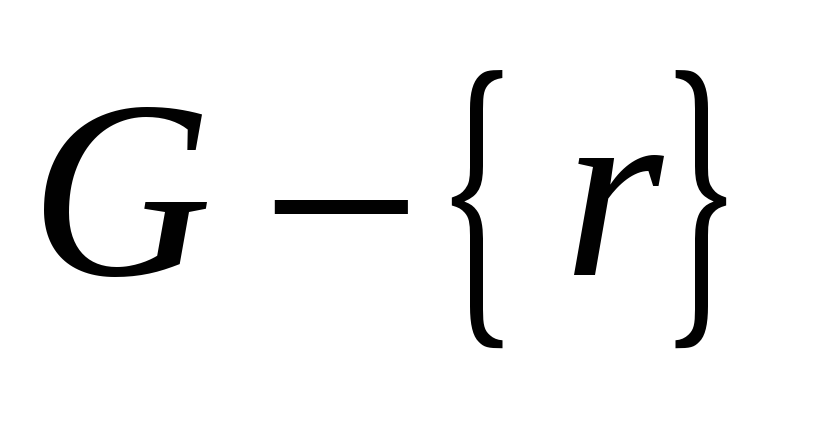. Thus prove that by means of a single minimum spanning tree computation, the k-tree problem can be reduced to a k-tree problem on a subgraph G′ of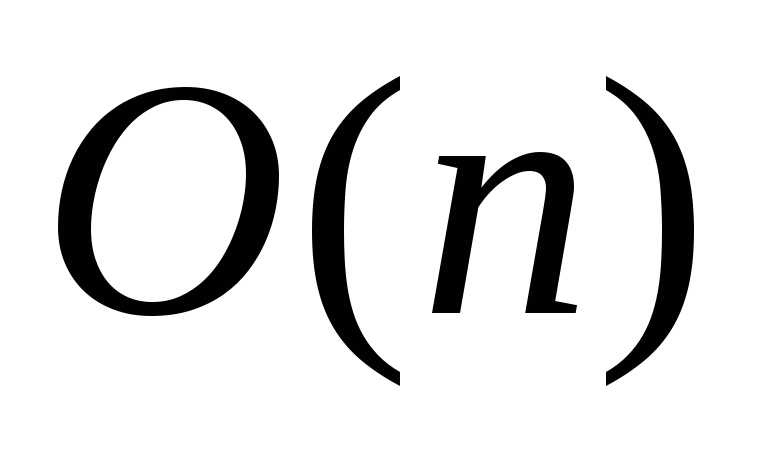edges, consisting of the union of a tree and the set of edges incident to r. Prove that a minimum k-tree can be obtained from a minimum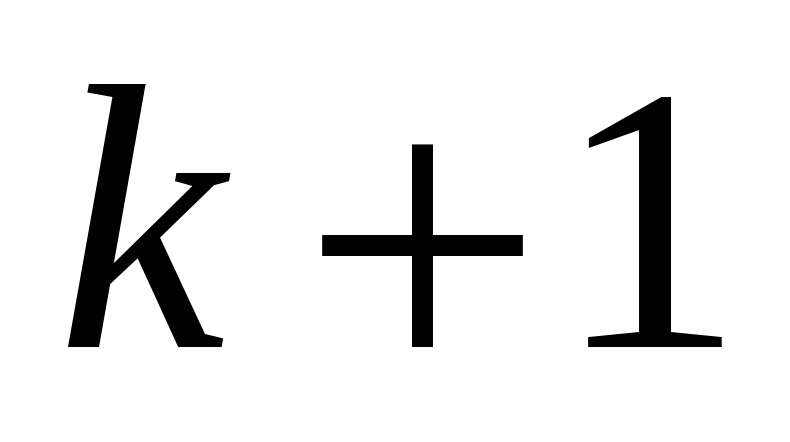(or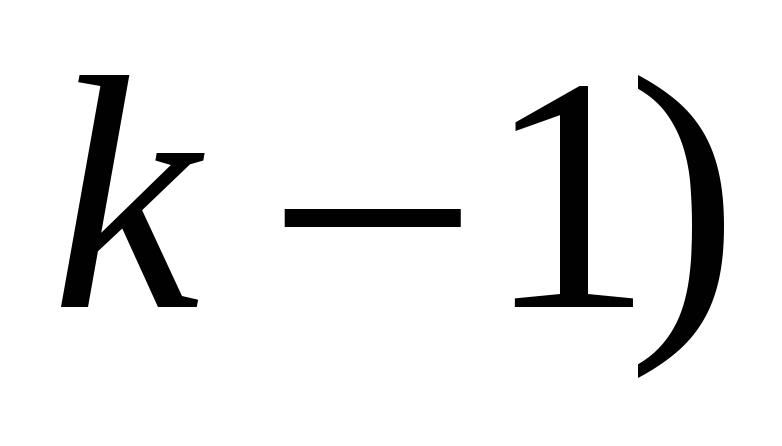tree by doing a single edge swap (adding one edge and deleting one other edge). Using this result, show how to find a minimum k-tree in G′ fast. (A minimally acceptable solution is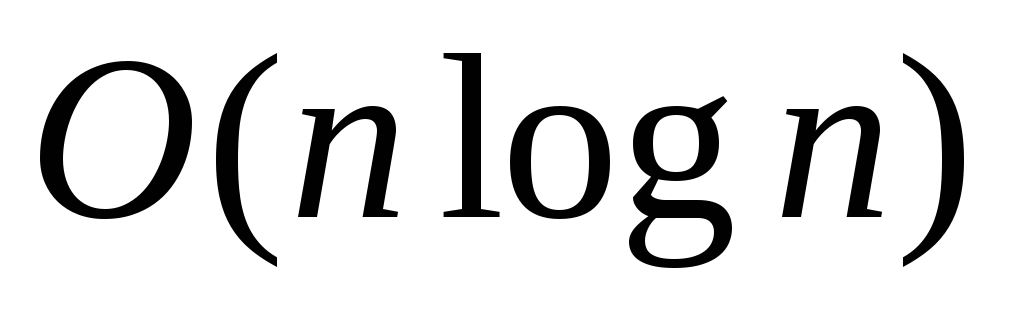time; there is a way to do it intime.) Download 0.51 Mb.Share with your friends: